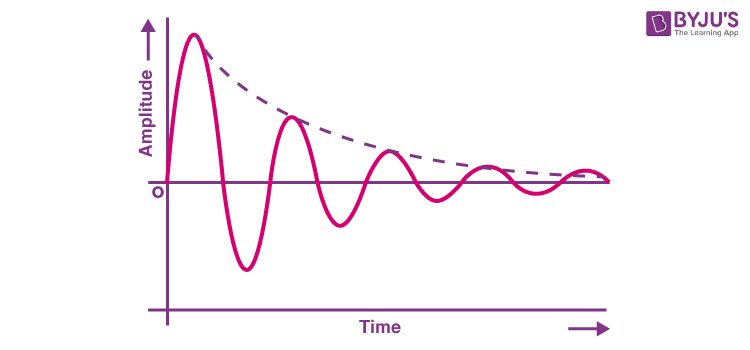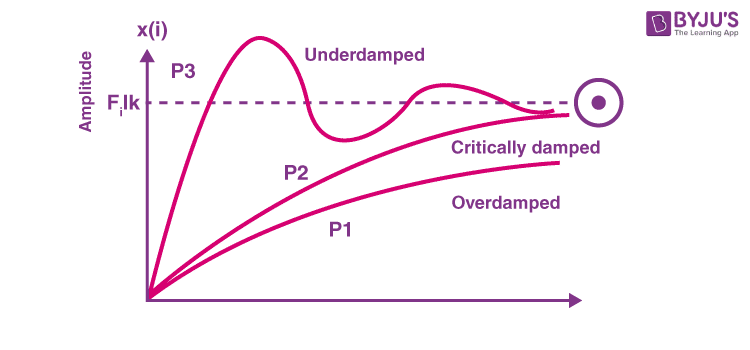Checkout JEE MAINS 2022 Question Paper Analysis : Checkout JEE MAINS 2022 Question Paper Analysis :

# Oscillation

## What is Oscillation?

Oscillation is defined as the process of repeating variations of any quantity or measure about its equilibrium value in time. Oscillation can also be defined as a periodic variation of a matter between two values or about its central value.

The term vibration is used to describe the mechanical oscillations of an object. However, oscillations also occur in dynamic systems or more accurately in every field of science. Even the beating of our heart creates oscillations​. Meanwhile, objects that show motion around an equilibrium point are known as oscillators.

## Examples of Oscillations

Most common examples for oscillation are the tides in the sea and the movement of a simple pendulum in a clock. Another example of oscillation is the movement of spring. The vibration of strings in guitar and other string instruments are also examples of oscillations.

The pendulum moves back and forth and hence it creates an oscillating movement​. Mechanical oscillations are called vibrations. A particle being vibrated means it oscillates between two points about its central point.

Likewise, the movement of spring is also oscillation. The spring moves downward and then upward repeatedly and hence it produces an oscillating movement.

A sine wave is a perfect example of oscillation. Here the wave moves between two points about a central value. The height or the maximum distance that the oscillation takes place is called the amplitude and the time taken to complete one complete cycle is called the time period of the oscillation. Frequency is the number of complete cycles that occur in a second. Frequency is the reciprocal of the time period.

F = 1 / T

Where F is the frequency of oscillation

And T is the time period of the oscillation.

## Oscillation Motion

In simple terms, we can say that when an object is swinging from side to side in a mechanical system this movement can be termed as oscillation motion. In this type of motion, the potential energy usually changes to kinetic energy. An oscillation motion consists of one complete cycle.

## Oscillation Types

Here we will look at the different types of oscillations.

### Damped Oscillations

Damping the process of restraining or controlling the oscillatory motion, such as mechanical vibrations, by the dissipation of energy. An oscillation remains undamped when a restoring force equal to the restraining for is induced and hence the system oscillates with the same energy. When the restoring force is not applied the oscillation suddenly stops. And when the restoring force applied is less than​ the restraining force, damping is introduced.

Damped oscillations are classified according to the difference in energy between the restoring force applied and the restraining force acting. A damped oscillation is an oscillation which fades away with respect to time. That is the oscillations which reduce in magnitude with time.

An ideal oscillating system is not a damped oscillation. In an ideal case, the magnitude of the oscillations does not reduce with time and the amplitude remains the same. Suppose a child is swinging in a swing, unless the swing is being pumped by another child or any external force, the motion of the swing fades and slowly the swing stops. This process is due to the damping of the swing.

● Shock absorbers in vehicles are examples of damping devices that reduces the vibration of the vehicle.

An image showing damped oscillation is given below;In the above figure, the amplitude of the wave is being diminished with time. The amplitude of the wave gets reduced and such oscillations are called damped oscillation.

### Different Types of Damped Oscillations

Damped oscillations can mainly be classified into three types

• Under damped oscillations: Damping constant <1
• Critically damped oscillations: Damping constant = 1
• Over damped oscillations: Damping constant >1A graph showing various damping system is shown above and the three types of damping systems are discussed below.

#### Under Damped Oscillations

An underdamped oscillation reaches the zero value or the equilibrium point faster but oscillations occur across the equilibrium point one or more time. In underdamped oscillations, the damping constant is less than one.

In underdamped oscillations, the oscillation becomes stable very slowly. Under damped oscillations have least energy dissipation compared to other damping systems.

#### Critically Damped Oscillations

Critically damping is the damping in which the damping of the oscillation causes it to return to its equilibrium point fast and does not create any oscillations back and forth about that point. Critical damping is the damping in which the damping constant is equal to one.

Critical damping is the precise damping required for a system. In critical damping, the damping applied is such that the force available is just sufficient to bring the oscillation into equilibrium without any further back and forth movement (oscillations).

#### Over Damped Oscillations

The damping in which the oscillations reach equilibrium point slowly and do not create any oscillations across the equilibrium point is termed as overdamped oscillations. In overdamped oscillations, the damping constant is greater than one. Over damped oscillations have higher energy dissipation compared with other damping systems.

In overdamped oscillations, the damping applied is more than the required value of damping in order to bring the oscillations into rest or equilibrium point and hence the oscillations reach the equilibrium point very slowly compared with other damping methods. In this system, no further back and forth movement will occur across the equilibrium point.

### Undamped Oscillations

An undamped oscillation is an oscillation in which, when displaced from its equilibrium point, experiences a restoring force which is proportional to the displacement. So, in the undamped oscillation system, the magnitude of the oscillations never fades and the magnitude of the oscillation remains the same. An example of undamped oscillation is alternating current (AC Wave)

The magnitude of alternating current oscillates between two values across the equilibrium value, repeatedly and without any change in magnitude or time period. In alternating currents, no restraining force acts and the magnitude of the signal does not fade with respect to time and keeps on maintaining the same amplitude.

## Frequently Asked Questions on Oscillations

### What are the three types of oscillations?

Free Oscillation
Damped Oscillation
Forced Oscillation

### What is a free oscillation?

When the body oscillates with its own frequency without any external influence is called free oscillation.

### What are forced oscillations?

The body vibrating with a natural frequency starts vibrating with the frequency of the applied periodic force due to the presence of external force is called forced oscillations.

### What is an oscillatory motion?

The motion that repeats itself is called oscillatory or periodic motion.